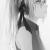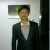百万数据如何在前端快速流畅显示？

justjavac 发布于 2014/03/31 13:55

一、 策略

```/==============> Data List |     ....     | /
+--------------+/ +=======|=====data=====|========+ |       +--------------+        | |       | data |        | |       +--------------+        |\ |       | data |        | \ |       +--------------+        |  \======> Container +=======|=====data=====|========+  +--------------+ |     ....     | Created By Barret Lee```

二、Demo

demo 在 IE 7、8 有 bug，请读者自己修复吧~

```<title>百万数据前端快速流畅显示</title> <style type="text/css"> #box {position: relative; height: 300px; width: 200px; border:1px solid #CCC; overflow: auto} #box div { position: absolute; height: 20px; width: 100%; left: 0; overflow: hidden; font: 16px/20px Courier;} </style> <div id="box"></div> <script type="text/javascript"> var total = 1e5 , len = total
, height = 300 , delta = 20 , num = height / delta
, data = []; for(var i = 0; i < total; i++){
data.push({content: "item-" + i});
} var box = document.getElementById("box");
box.onscroll = function(){ var sTop = box.scrollTop||0 , first = parseInt(sTop / delta, 10)
, start = Math.max(first - num, 0)
, end = Math.min(first + num, len - 1)
, i = 0; for(var s = start; s <= end; s++){ var child = box.children[s]; if(!box.contains(child) && s != len - 1){
insert(s);
}
} while(child = box.children[i++]){ var index = child.getAttribute("data-index"); if((index > end || index < start) && index != len - 1){
box.removeChild(child);
}
}

}; function insert(i){ var div = document.createElement("div");
div.setAttribute("data-index", i);
div.style.top = delta * i + "px";
div.appendChild(document.createTextNode(data[i].content));
box.appendChild(div);
}

box.onscroll();
insert(len - 1); </script>```

三、算法说明

1. 计算 start 和 end 节点

```|              |
+=======|==============|========+——
|    ↓——+--------------+        | ↑ | delta |              |        |
|    ↑——+--------------+        | height |       |              |        |
|       +--------------+        | ↓ +=======|==============|========+——
|              |```

Container 可以容纳的 Data 数目为 num = height / delta，Container 顶部第一个节点的索引值为

`var first = parseInt(Container.scrollTop / delta);`

`var start = Math.max(first - num, 0); var end = Math.min(first + num, len - 1);`

2. 插入节点

```// 插入最后一个节点 insert(len - 1); // 插入从 start 到 end 之间的节点 for(var s = start; s <= end; s++){
var child = Container.children[s];
// 如果 Container 中已经有该节点，或者该节点为最后一个节点则跳过
if(!Container.contains(child) && s != len - 1){ insert(s); }
}```

```function insert(i){
var div = document.createElement("div");
div.setAttribute("data-index", i);
div.style.top = delta * i + "px";
div.appendChild(document.createTextNode(data[i].content));
Container.appendChild(div);
}```

3. 删除节点

```while(child = Container.children[i++]){
var index = child.getAttribute("data-index"); // 这里记得不要把最后一个节点给删除掉了 if((index > end || index < start) && index != len - 1){
Container.removeChild(child);
}
}```

四、小结

00